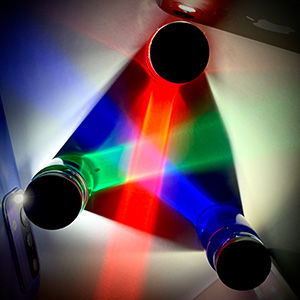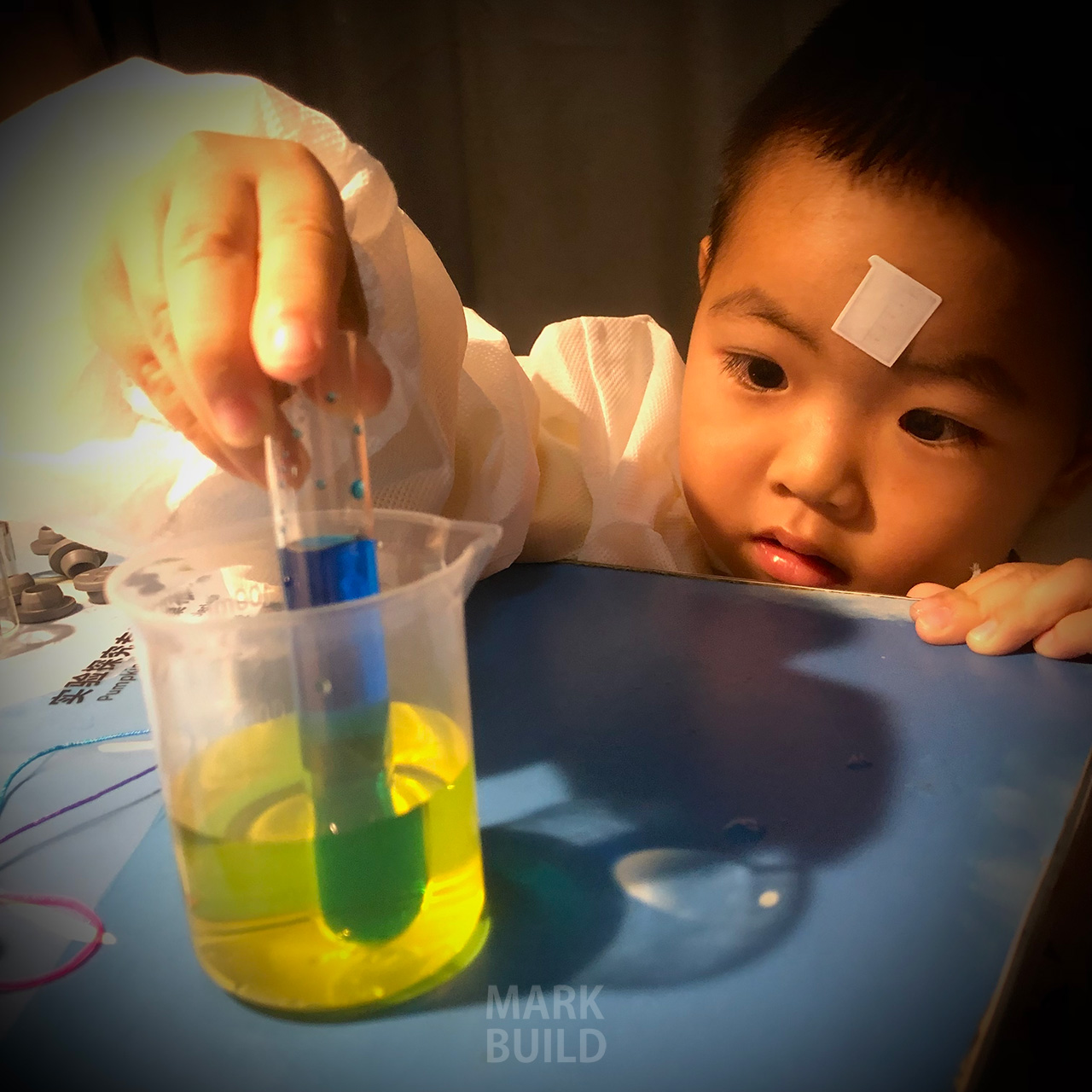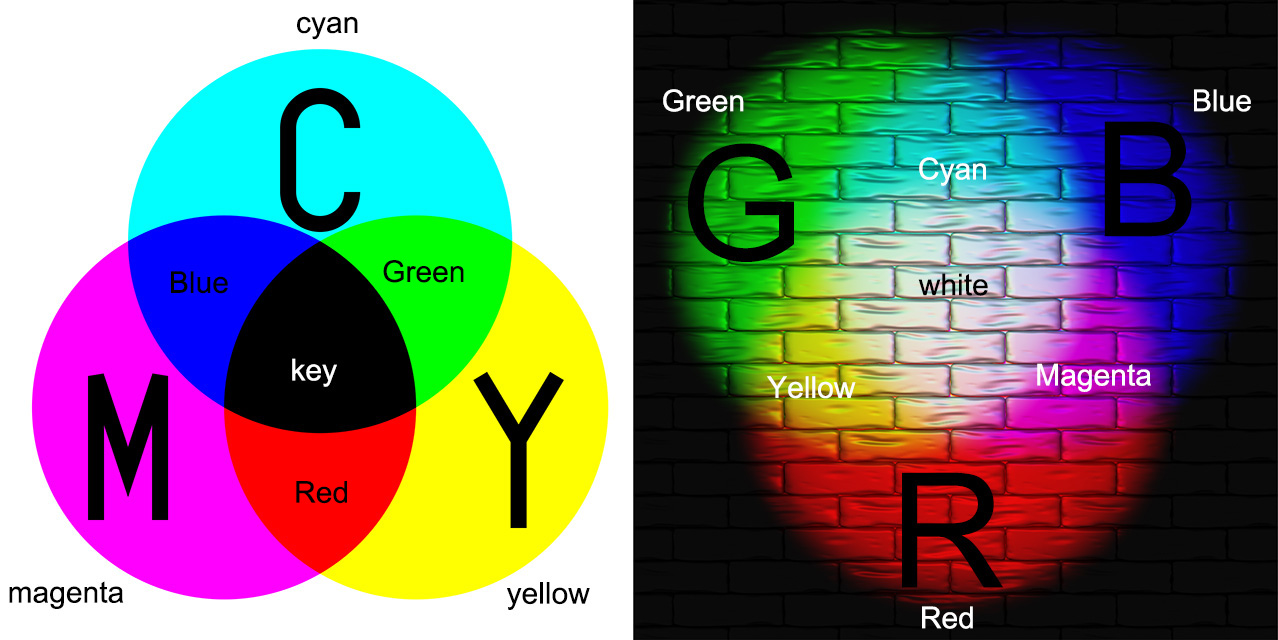# 颜色混合及 RGB 与 CMYK 颜色蓝+黄=绿Magenta + Cyan = Blue 洋红 + 青 = 蓝Magenta + Yellow = Red 洋红 + 黄 = 红Cyan + Yellow = Green 青 + 黄 = 绿Green + Red = Yellow 绿 + 红 = 黄Green + Blue = Cyan 绿 + 蓝 = 青Red + Blue = Magenta 红 + 绿 = 洋红

## 什么是CYMK 颜色模型

CYMK 颜色模型是一种减色模型，比如颜料混合。CMYK 模型的工作原理是在较浅（通常是白色）的背景上部分或完全遮盖颜色。墨水减少了否则会被反射的光，从白光中“减去”红色、绿色、蓝色。白光减红色呈现青色，白光减绿色呈现品红，白光减蓝色呈现黄色。

## 参考引用

https://en.wikipedia.org/wiki/CMYK_color_model
https://en.wikipedia.org/wiki/RGB_color_model
https://en.wikipedia.org/wiki/Spectral_power_distribution